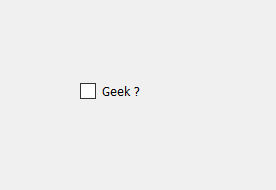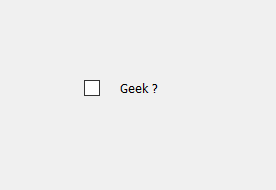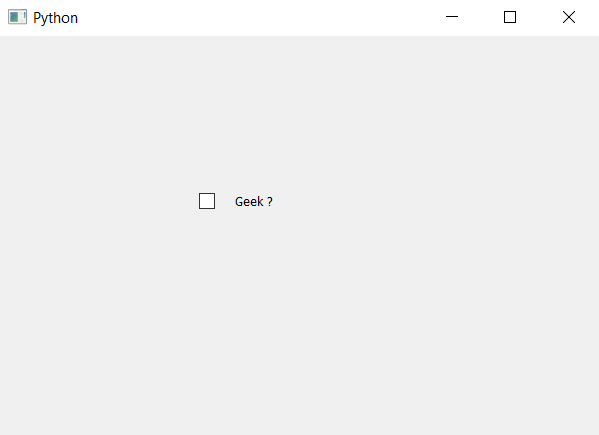# PyQt5 – Add spacing between indicator and Check box

• Last Updated : 19 May, 2022

In this article we will see how to set spacing in between the check box and the indicator. When we create a check box by default some space in between them, but we change that space also. Below is the representation of default check box vs the increased spaced check box.This can be done by changing the size of spacing in the style sheet of check box, below is the style sheet code which is used with check box object.

```QCheckBox
{
spacing : 20px;
}```

Below is the implementation.

## Python3

 `# importing libraries``from` `PyQt5.QtWidgets ``import` `*``from` `PyQt5 ``import` `QtCore, QtGui``from` `PyQt5.QtGui ``import` `*``from` `PyQt5.QtCore ``import` `*` `import` `sys`  `class` `Window(QMainWindow):` `    ``def` `__init__(``self``):``        ``super``().__init__()` `        ``# setting title``        ``self``.setWindowTitle("Python ")` `        ``# setting geometry``        ``self``.setGeometry(``100``, ``100``, ``600``, ``400``)` `        ``# calling method``        ``self``.UiComponents()` `        ``# showing all the widgets``        ``self``.show()` `    ``# method for widgets``    ``def` `UiComponents(``self``):` `        ``# creating the check-box``        ``checkbox ``=` `QCheckBox(``'Geek ?'``, ``self``)` `        ``# setting geometry of check box``        ``checkbox.setGeometry(``200``, ``150``, ``100``, ``30``)` `        ``# adding spacing in between indicator and check box``        ``checkbox.setStyleSheet("QCheckBox"``                               ``"{"``                               ``"spacing : ``20px``"``                               ``"}")`   `# create pyqt5 app``App ``=` `QApplication(sys.argv)` `# create the instance of our Window``window ``=` `Window()` `# start the app``sys.exit(App.``exec``())`

Output :My Personal Notes arrow_drop_up